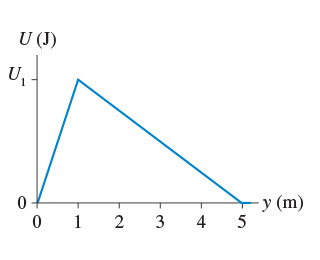# Problem: A system in which only one particle can move has the potential energy shown in  . Suppose U1 = 80 J.(a) What is the y-component of the force on the particle at y = 0.5 m ?(b) What is the y-component of the force on the particle at y = 4 m?

###### FREE Expert Solution

We are given the potential energy graph of a particle. The relationship between force and potential energy is:

Force:

$\overline{){\mathbf{F}}{\mathbf{=}}{\mathbf{-}}\frac{\mathbf{∆}\mathbf{U}}{\mathbf{∆}\mathbf{y}}}$

80% (132 ratings)###### Problem Details

A system in which only one particle can move has the potential energy shown in. Suppose U1 = 80 J.

(a) What is the y-component of the force on the particle at y = 0.5 m ?

(b) What is the y-component of the force on the particle at y = 4 m?

Frequently Asked Questions

What scientific concept do you need to know in order to solve this problem?

Our tutors have indicated that to solve this problem you will need to apply the Force & Potential Energy concept. You can view video lessons to learn Force & Potential Energy. Or if you need more Force & Potential Energy practice, you can also practice Force & Potential Energy practice problems.

What professor is this problem relevant for?

Based on our data, we think this problem is relevant for Professor Wang's class at GSU.